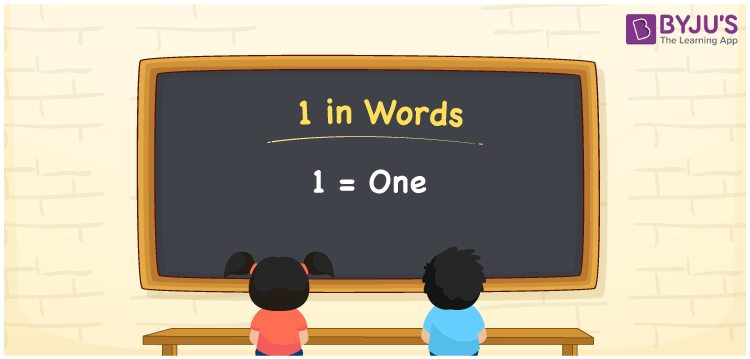# 1 in Words

1 in words is One. To represent 1 in words, we should know how to read and write in English since it is the most widely used language in the field of Education. We know that 1 is a cardinal number as it denotes a specific value of something. Learn to write the number 1 in English as well as how to spell it in this article.

 1 in words One One in numerical form 1

## 1 in English Words

Generally, we use the English alphabet to write numbers in words. Students must know how to read and write in English since it is the most used language. Hence, 1 in words is written as One.## How to Write 1 in Words?

Using the place value of a number, we can write the number 1 in words. The below table represents the place value chart for the number 1.

 Tens Ones 0 1

Hence, we can write the expanded form as:

0 × Ten + 1 × One

= 0 x 10 + 1 x 1

= 0 + 1

= 1

= One

Thus, 1 in words is written as One

Interesting way of writing 1 in words

0 = Zero

1 = One

0 + 1 = 1

1 = One

Zero + One = One

Hence, the word form of the number 1 is One

1 is a natural number that is succeeded by 0 and preceded by 2

• 1 in words – One
• Is 1 an odd number? – Yes
• Is 1 an even number? – No
• Is 1 a perfect square number? – Yes
• Is 1 a perfect cube number? – Yes
• Is 1 a prime number? – No
• Is 1 a composite number? – No

## Frequently Asked Questions on 1 in Words

Q1

### How to write 1 in words?

1 in words is written as One.
Q2

### Is 1 a perfect square number?

Yes, 1 is a perfect square number.
Q3

### Write One in numbers?

One in numbers is 1.
Test your Knowledge on 1 in Words